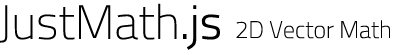Node Parcel Merchant

# npm

## justmath

1.0.1 • Public • Published

#An implementation of two dimensional vector math including a rich toolset for vector operations. It's also the math library behind eSoccer, a cross-platform multiplayer HTML5 game developed at University of Applied Sciences Bonn.

## JustMath

• Augments core Math
• Allows replacement of all methods (e.g. custom implementations of `JustMath.sqrt(value)`)
• Adds some convenience methods (`JustMath.sq(value)`, `JustMath.cot(angle)`)

## JustMath.Vec2

• Vector instantiation (`new Vec2(vOrX[, y])`) and cloning (`Vec2#clone()`)

• Direct modification through `Vec2#x` and `Vec2#y`, also provides getters (`Vec2#getX()`, `Vec2#getY()`) and a setter (`Vec2#set(vOrX[, y])`)

• Vector addition (`Vec2#add(vOrX[, y])`), subtraction (`Vec2#sub(vOrX[, y])`) and multiplication (`Vec2#dot(vOrX[, y]))`)

• Vector orthogonality (`Vec2#ort()`)

• Vector normalization (`Vec2#norm()`), scaling (`Vec2#scale(factor)`), inversion (`Vec2#inv()`) and magnitude (`Vec2#mag()`, `Vec2#magSq()`) calculation

• Vector-Vector distances (`Vec2#dist(b)`, `Vec2#distSq(b)`)

• Vector rotation (`Vec2#rot(angle)`) and direction calculation (`Vec2#dir()`)

• Vector projection (`Vec2#project(b)`) and rejection (`Vec2#reject(b)`)

• Vector reflection (`Vec2#reflect(n)`), also with component-wise (projected and rejected component) scaling (`Vec2#reflectAndScale(n, projectFactor, rejectFactor)`)

• Vector interpolation (`Vec2#lerp(p, percent)`)

• Vector containment in rectangle (`Vec2#inRect(p1, p2)`)

• [Vector,Vector] determinant calculation (`Vec2.det(v1, v2)`)

• Provides Vector#toString and `Vector#equals()`

• Provides vector operation chaining, e.g.

• Provides `Vec2#toString()` for pain-free debugging

• Is of course able to evaluate `Vec2#equals(b)`

• Exports and imports JSON payloads (`Vec2#getXY()`, `new Vec2(jsonPayload)`)

• Small allocation footprint when using `Vec2#clone()` wisely

• Accepts another Vec2 or plain X and Y coordinates as parameters where possible (e.g. `Vec2#add(vOrX[, y])`)

## Features

• CommonJS compatible
• RequireJS/AMD compatible
• Shim compatible
• node.js compatible, also available via npm (npm install justmath)
• Closure Compiler ADVANCED_OPTIMIZATIONS compatible (fully annotated)
• Fully documented using jsdoc3
• Zero dependencies and prerequisites
• Small footprint

## Usage

### Node.js / CommonJS

• Install: `npm install justmath`

## Keywords

none

### Install

`npm i justmath`

### Repository

github.com/dcodeIO/JustMath.js